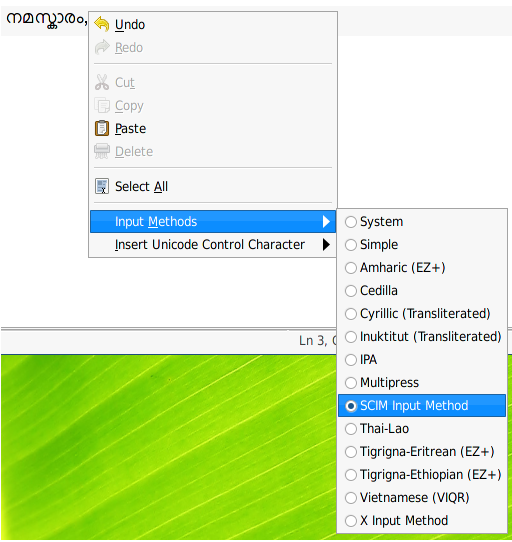# A simple square pattern in C++

Here's a small C++ program which outputs a square pattern according to the size entered. Code is given below :

[sourcecode language="cpp" light="true"]

/*
* sqpattern.cpp
*
* Licensed under GPL Version 3
*
* To compile - g++ -o sqpattern sqpattern.cpp
* To execute - ./sqpattern
*/

#include <iostream>
using namespace std;

int main()
{
int size;
cout << "Enter size: ";
cin >> size;

int count = 1;
cout << " ";
while (count++ < size) cout << " _";

for (int i = 1; i < size; i++)
{
int numOfCharsPerLine = 0;
cout << "n";
for (int j = 0; j < i; j++)
{
cout << " |";
numOfCharsPerLine += 2;
}

cout << "_";
numOfCharsPerLine += 1;

int k = 1;
while (k++ < (size*2) - numOfCharsPerLine )
cout << " ";
cout << "|";
}

count = 1;
cout << "n ";
while (count++ <= (size)-1 ) cout << "|_";

cout << "|n";

return 0;
}

[/sourcecode]

Output:The programs draws  squares of any size entered. The code needs improvement, please feel free to give suggestions. Click here for 'experiments' git repo. Thank you :)

Tags:

Categories: